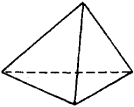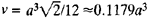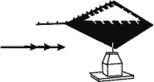tetrahedron

(redirected from 3-simplex)
Also found in: Dictionary, Thesaurus.

tetrahedron:

see polyhedronpolyhedron
, closed solid bounded by plane faces; each face of a polyhedron is a polygon. A cube is a polyhedron bounded by six polygons (in this case squares) meeting at right angles.
.

Tetrahedron

A polygon with four plane surfaces.
The following article is from The Great Soviet Encyclopedia (1979). It might be outdated or ideologically biased.

Tetrahedron

(or triangular pyramid), a polyhedron with four triangular faces, six edges, and four vertices. At each vertex three edges intersect. The regular tetrahedron (Figure 1) is oneFigure 1

of the five regular polyhedrons. If a is the length of an edge of a regular tetrahedron, the volume of the tetrahedron is.

tetrahedron

[‚te·trə′hē·drən]
(crystallography)
An isometric crystal form in cubic crystals, in the shape of a four-faced polyhedron, each face of which is a triangle.
(mathematics)
A four-sided polyhedron.
McGraw-Hill Dictionary of Scientific & Technical Terms, 6E, Copyright © 2003 by The McGraw-Hill Companies, Inc.

tetrahedronA device to indicate wind direction, and, in turn, landing direction. It is tetrahedronshaped—four triangular sides. This device is generally located at uncontrolled airports. The small end of a tetrahedron points in the direction of landing. At controlled airports, the tetrahedron should be disregarded because tower instructions supercede the indicator. On approach charts, a tetrahedron is shown as.